# Find the volume of the solid that lies inside the sphere 2 + y2 + 2...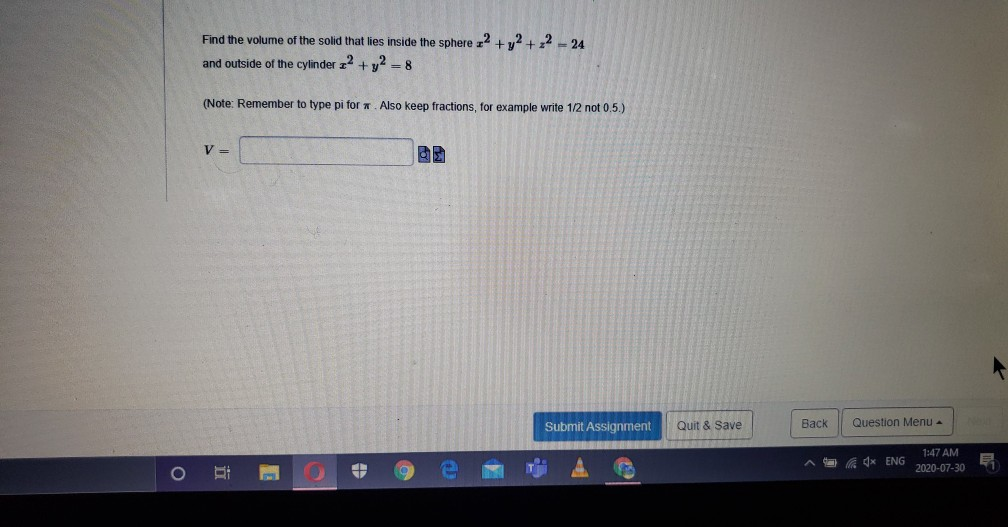Find the volume of the solid that lies inside the sphere 2 + y2 + 2 - 24 and outside of the cylinder 2 + y2 = 8 (Note: Remember to type pi for. Also keep fractions, for example write 1/2 not 0.5.) V Submit Assignment Quit & Save Back Question Menu - Ad* ENG 1:47 AM 2020-07-30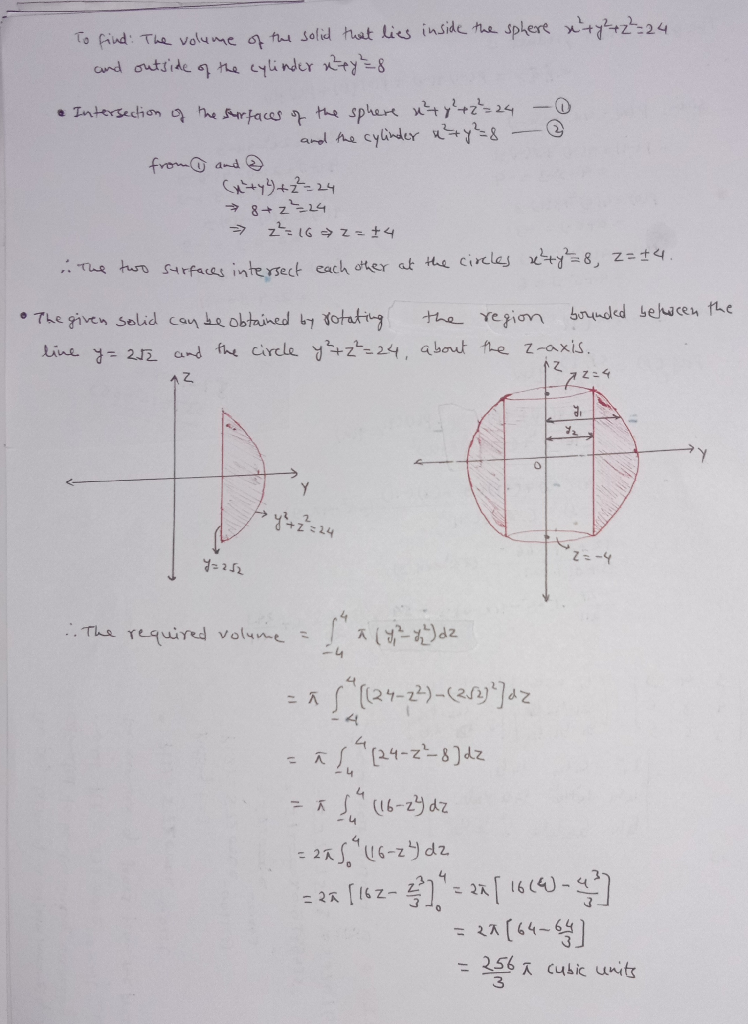##### Add Answer of: Find the volume of the solid that lies inside the sphere 2 + y2 + 2...
Similar Homework Help Questions
• ### Find the volume of the solid bounded by the cylinder z2 + y2 =1, and the...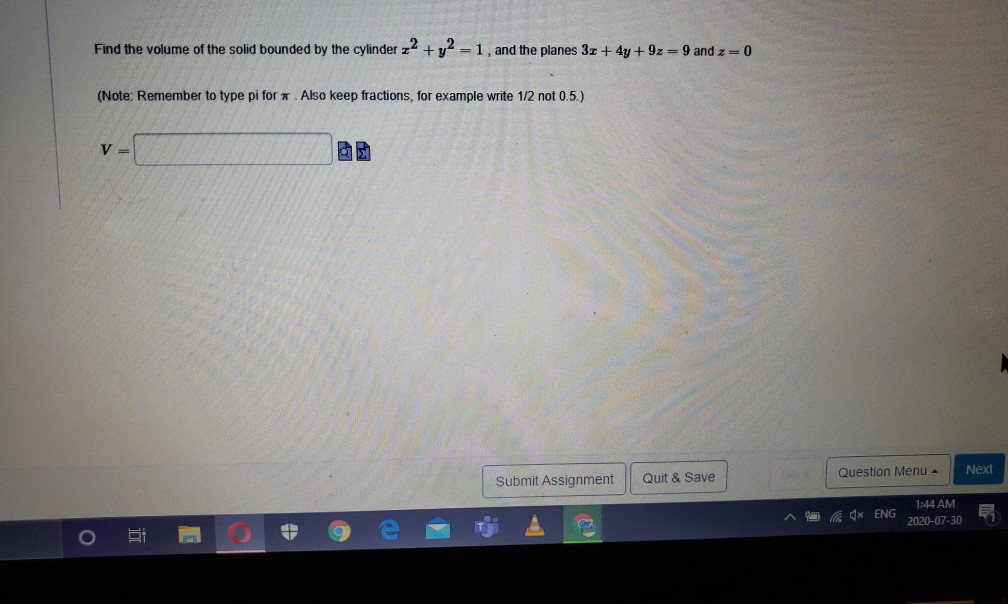Find the volume of the solid bounded by the cylinder z2 + y2 =1, and the planes 3 + 4y +9z=9 and z=0 (Note: Remember to type pi for. Also keep fractions, for example write 1/2 not 0.5.) V= Next Submit Assignment Quit & Save Question Menu 4x ENG 1:44 AM 2020-07-30

• ### Find the volume of the solid that lies inside the sphere x2 + y2 + 2...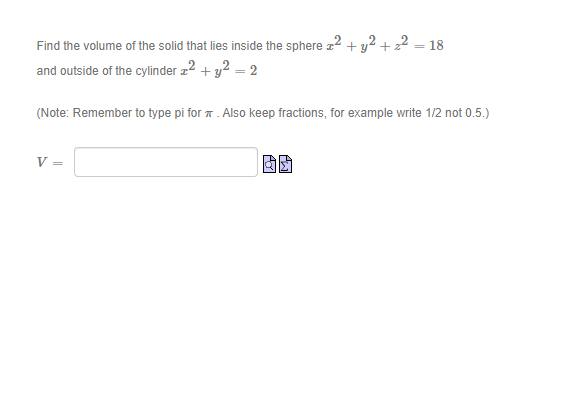Find the volume of the solid that lies inside the sphere x2 + y2 + 2 = 18 and outside of the cylinder 22 + y2 = 2 (Note: Remember to type pi for . Also keep fractions, for example write 1/2 not 0.5.) V=

• ### er 20 / Quiz 9 Remaining Time: 138:14 Evaluate 8 +y2 +22)-1/2 av where D is...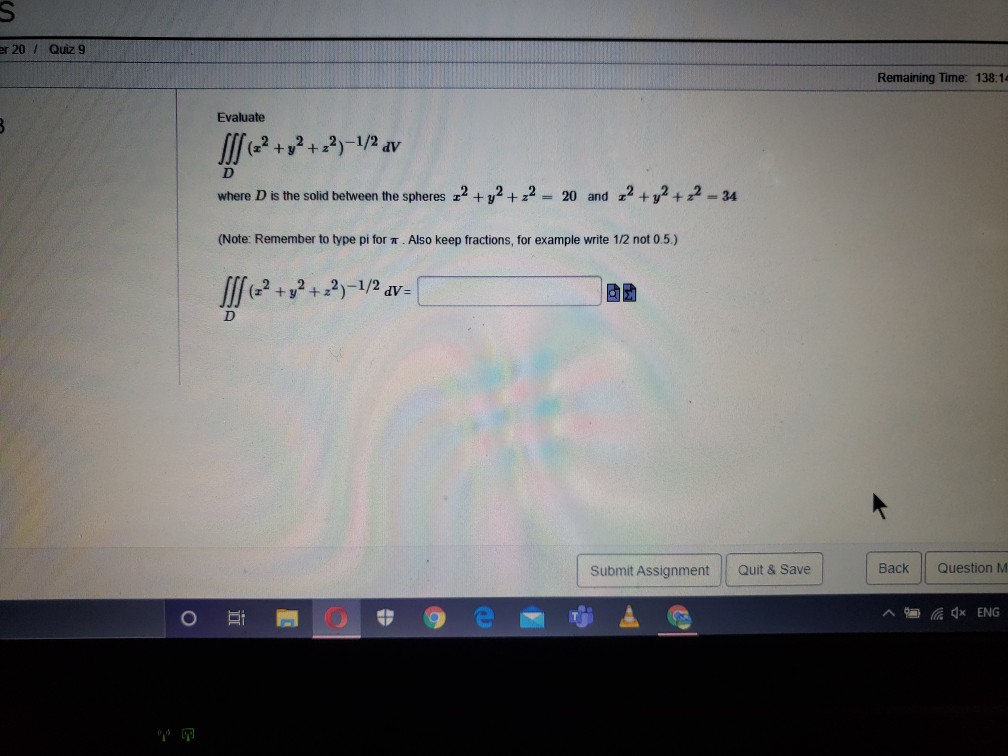er 20 / Quiz 9 Remaining Time: 138:14 Evaluate 8 +y2 +22)-1/2 av where D is the solid between the spheres 22 + y2 + 2 = 20 and 2 + y2 + 2 – 34 (Note: Remember to type pi for. Also keep fractions, for example write 1/2 not 0.5.) IS + y2 +22)-1/2 dv= D Submit Assignment Quit & Save Back Question M O 4x ENG

• ### Find the volume of the solid bounded by the cylinder x2 + y2 = 1, and...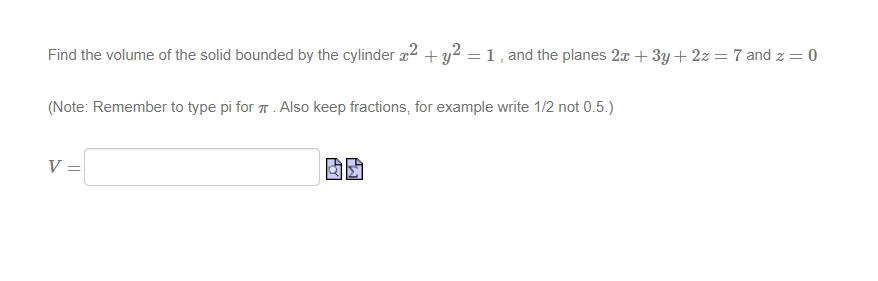Find the volume of the solid bounded by the cylinder x2 + y2 = 1, and the planes 2x + 3y + 2z = 7 and 2 = 0 (Note: Remember to type pi for 7. Also keep fractions, for example write 1/2 not 0.5.) V= M

• ### (1 point) Find the volume of the solid that lies within the sphere x2 +y2 .2 16, above the xy plane, and outside the co...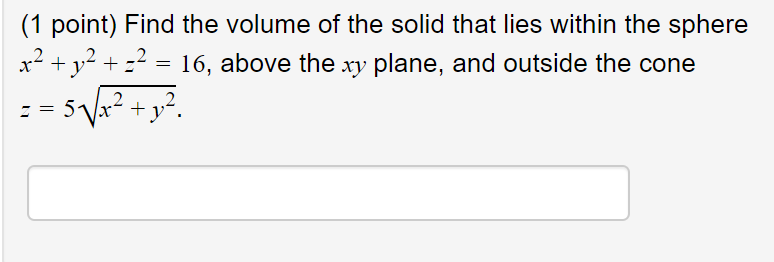(1 point) Find the volume of the solid that lies within the sphere x2 +y2 .2 16, above the xy plane, and outside the cone 2 (1 point) Find the volume of the solid that lies within the sphere x2 +y2 .2 16, above the xy plane, and outside the cone 2

• ### (1 point Find the volume of the solid that lies within the sphere x2 + 2 + z-64 above the xy plane, and outside the cone z 8V x2 y2 (1 point Find the volume of the solid that lies within the sph...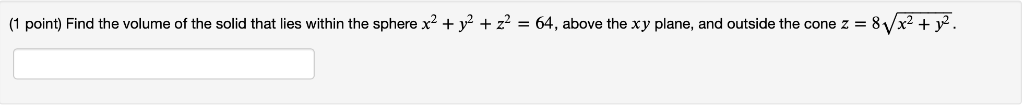(1 point Find the volume of the solid that lies within the sphere x2 + 2 + z-64 above the xy plane, and outside the cone z 8V x2 y2 (1 point Find the volume of the solid that lies within the sphere x2 + 2 + z-64 above the xy plane, and outside the cone z 8V x2 y2

• ### you can choose more than one answer! Question 2 Suppose you have to use spherical coordinates...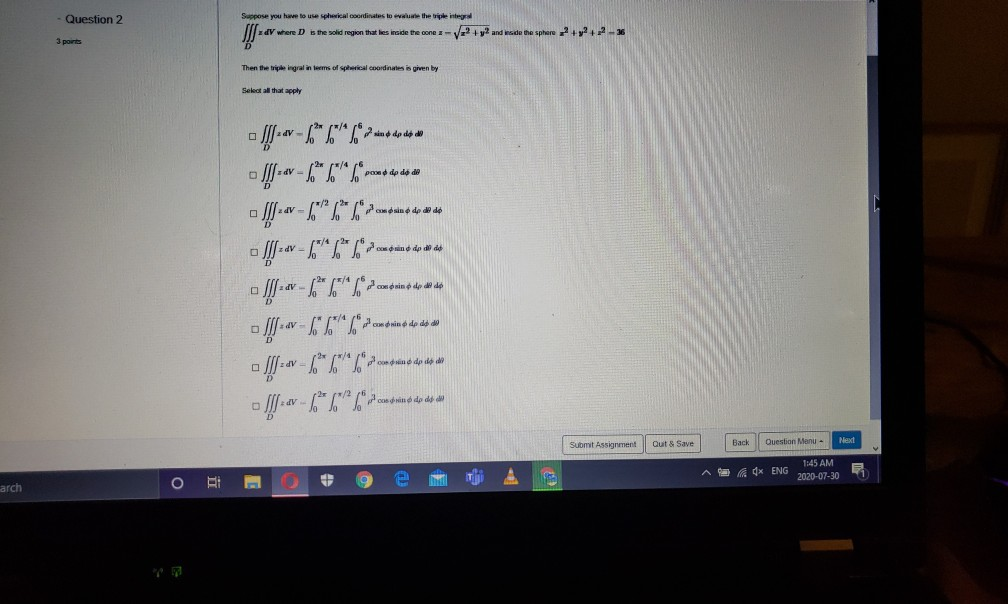you can choose more than one answer! Question 2 Suppose you have to use spherical coordinates to the triple integy where is the solid region that lies incide the cone - V2 + y2 and wade the sphere 2+2+2-36 3 ports Then the triple ingral in terms of spical coordinates is given by Select all that apply *****,)..1-1 *****0,7.1.1-x-fift ******).T/9---fff *******<,)1,9---ff- - A---6"L" /dcbi* * * av - "L" /can din é do dys - [--- 6"""" povremo to use...

• ### volume of a solid bounded by sphere & coneFind the volume of the solid bounded inside the sphere x2 + y2 + z2 =9 and outside thecone z= .Be sure to sketch – note in particular that negative gives a cone that lies below the plane.I am validating my work so please show your steps so I can determine where/if I went wrong.

• ### 2. (20 pts) Find the surface area of that part of the sphere x2 + y2 + z2-4 that lies inside the paraboloid z x2 + y2. 2. (20 pts) Find the surface area of that part of the sphere x2 + y2 +...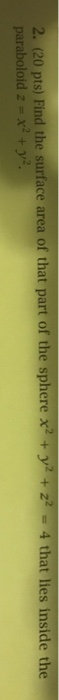2. (20 pts) Find the surface area of that part of the sphere x2 + y2 + z2-4 that lies inside the paraboloid z x2 + y2. 2. (20 pts) Find the surface area of that part of the sphere x2 + y2 + z2-4 that lies inside the paraboloid z x2 + y2.

• ### Use polar coordinates to find the volume of the given solid. Inside the sphere and outside...

Use polar coordinates to find the volume of the given solid. Inside the sphere and outside the cylinder = 25 We were unable to transcribe this image = 25

Free Homework App